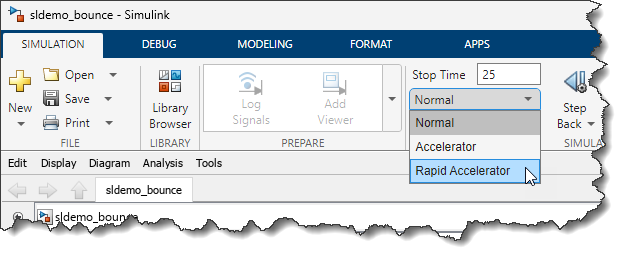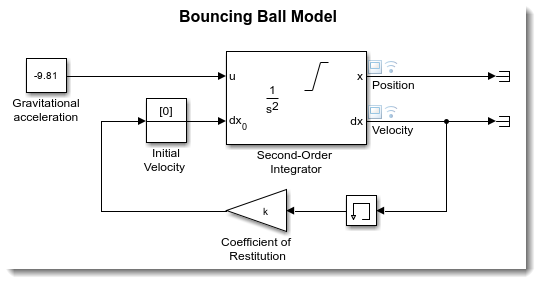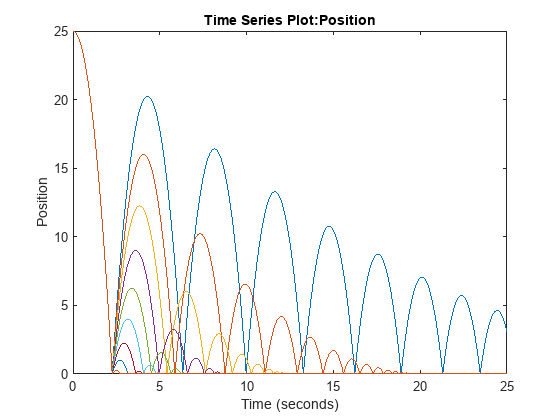# Getting the most out of Rapid Accelerator mode – Version R2023b

Earlier this week, a user came to me with a question related to a blog post I wrote a long time ago: Getting the most out of Rapid Accelerator mode. This made me realize that since then, we have made significant usability improvements to this workflow. This inspired me to rewrite this blog post using the latest recommended syntax as of MATLAB R2023b.

## Rapid Accelerator

As explained here, the Rapid Accelerator simulation mode can speed up simulation by generating an executable for your model. The speedup can vary between models and for various reasons. Personally, I have seen some models run more than 10 times faster in Rapid Accelerator mode.## RapidAcceleratorUpToDateCheck = off

When you click the play button in a model or use the sim command, Simulink checks if your model has changed or not since the last simulation. If the model has not changed structurally, the Rapid Accelerator executable is not re-generated. Obviously, verifying if the model has changed requires some work and can take a non-negligible amount of time for large models.
To help with that, Rapid Accelerator has an option to skip this check and directly use the already existing executable, if one already exists.
Let's see how this can be done using a simple demo like sldemo_bounce. For this example, we will vary the coefficient of restitution, specified by variable k used in a Gain block.Let's define a vector of coefficients we want to simulate for:
k_values = [-0.9:0.1:-0.1];
Then we create a vector of Simulink.SimulationInput objects that will tell Simulink to:
• Simulate the model in Rapid Accelerator mode
• Skip the up-to-date check
• Run one simulation per coefficient of restitution value
mdl = 'sldemo_bounce';
for i = 1:length(k_values)
in(i) = in(i).setModelParameter('SimulationMode','Rapid');
in(i) = in(i).setModelParameter('RapidAcceleratorUpToDateCheck','off');
in(i) = in(i).setVariable('k',k_values(i));
end
And that's it, we can simulate the model for all those values:
out = sim(in,'ShowProgress','off');
(Note: If you want the simulations to be executed in parallel, you can simply replace sim with parsim)
At the end, an array of Simulink.SimulationOutput objects is generated, from which we can extract and plot the results of each simulation.
for i = 1:length(out)
plot(out(i).logsout.get('Position').Values)
hold on;
end## What if I modified the model?

I mentioned it before, but I want to emphasize it here: With the RapidAcceleratorUpToDateCheck option set to off, Simulink will not check if your model has changed. It's up to you to tell Simulink to verify if the model has changed and to rebuild it if needed using Simulink.BlockDiagram.buildRapidAcceleratorTarget.
In this case, I have not modified the model, so Simulink.BlockDiagram.buildRapidAcceleratorTarget does not rebuild it and informs me that it was already up to date.